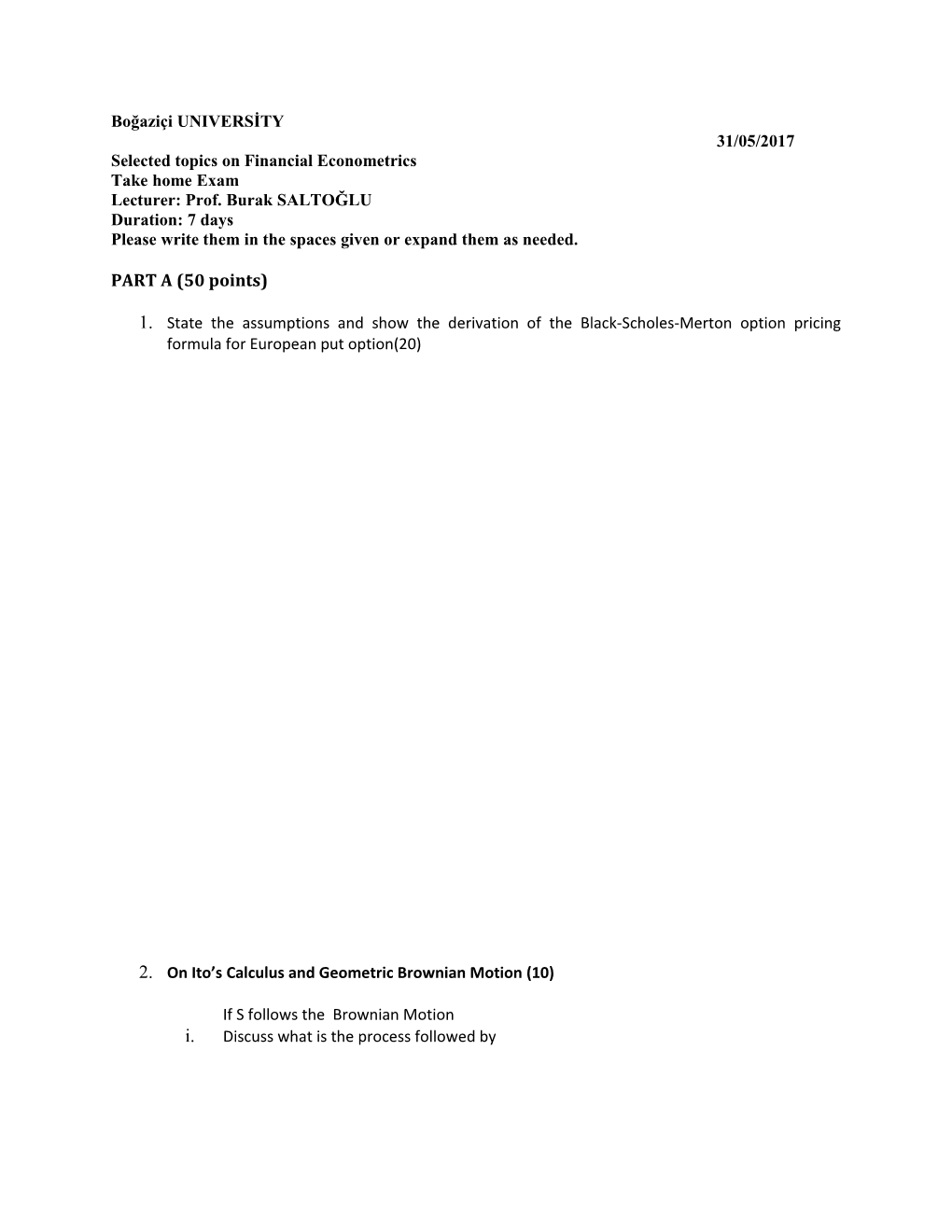# Selected Topics on Financial EconometricsBoğaziçiUNIVERSİTY 31/05/2017

Selected topics on Financial Econometrics

Take home Exam

Lecturer: Prof. Burak SALTOĞLU

Duration: 7 days

Please write them in the spaces given or expand them as needed.

PART A (50 points)

1. State the assumptions and show the derivation of the Black-Scholes-Merton option pricing formula for European put option(20)
1. On Ito’s Calculus and Geometric Brownian Motion (10)

If S follows the Brownian Motion

1. Discuss what is the process followed by

3.)(10) Suppose the last 250 days of returns of an Exchange Rate are reordered in an ascending order. The ordered returns are {-8,-6,-3.5,-3.4,-3,-3,-2,-1,-1,-1...... ,+2,+6,+8,+9}.

a.)Estimate the 1% VaR for a long position ? How can you estimate the VaR of 1% of a short position (i.e. if you have borrowed in this currency)?

b.) Further suppose that the daily return volatility is estimated to be 2%. How do you compare your VaR figure in a and b? What can we say about the skewness property of this return series

c.) At the same time the implied volatility (measured from at-the-money options with 60 days maturity) is 25% per year. What can be said about the future uncertaninty. Would you look for additional information to get a better forecast accuracy?

4. (10) Suppose for the Turkish FX market (USD/TR) we use the following GARCH(1,1) ( volatility model which has the following coefficient estimates: . (Note that epsilon and returns are treated in the same way with AR(0) assumption).

1. find the (yearly) long term volatility level.

b. if the most recent return and volatility levelsare ( ) respectively what would be the next day daily volatility forecast. Next week’s (15 days ahead) volatility forecast. (is it different than that of square root model i.e. Wiener Process assumptions).

c.Estimate the same model GARCH with the most recent data and compare your results..

1. Reserve the last 10% of your sample for volatiltiy forecasting. How does your model behave.
1. If we expect that Turkish FX series carry long memory property would the above model be adequate or not.

PART B (50)

İn the following you are given the USD/TL option for 3 and 6 months respectively.

1. Find their respective, delta, gamma, vega and rho’s of these two options.c.) In both cases first calculate the forward usd/TL for 3 and 6 months of maturity of contracts. Show that you find similar results with bloomberg. Then find the forex implied forward TL interest rate by using USD interest rate given. Discuss why Forward implied TL rate is useful in the Turkish banking sector.

d. set up a covered call position on the 31 st of may. Then calculate how much money you can make during the exam period.

e.. set up a delta hedge and use dynamic hedging in strategiesstarting from the 31st of may until 5 june. Just change the spot and forward USD/TLrates and keep the volatilty and interest rates constant. Step by step explain how you do the dynamic hedging and how much you can make money.

f..is there a need to do gamma hedge? . İf yes how can we conduct a gamma hedge..Show what options can be bought and how can you manage your gamma hedge

g.. if you were an importer how would you do the forex hedging?

h. In both of the options derive the implied volatilities. What is the historical volatility for USD/trl in 31st of May.

iIn the second half of 2017 FED is considering to increase the short rates of interest (which may be followed by many other central banks). Under this conditions what type of option trading strategies you may consider. Give 2 alternative option trading strategies with reasons and calculations. What is your preferred timing to enter a position?What levels of volatility could be a good point to start?

Useful formula